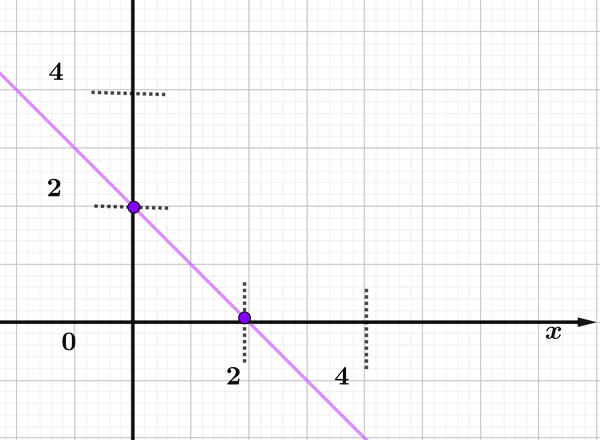Math Calculators, Lessons and Formulas

It is time to solve your math problem

mathportal.org
• Linear inequalities
• Solving linear equations and inequalities
• Point-slope form

# Point-slope form

ans:
syntax error
C
DEL
ANS
±
(
)
÷
×
7
8
9
4
5
6
+
1
2
3
=
0
.
auto next question
calculator
•  Question 1: 1 pts Point-slope is the general form $y+y_{1}=m(x+x_{1})$ for linear equations.
•  Question 2: 1 pts Write an equation in point-slope form of the line that passes through the point $(7,-6)$ and has the slope $m=6.$
 $y=-6x+36$ $y+6=6(x-7)$ $y-6=-3(x+7)$ $y=6x-42$
•  Question 3: 1 pts Write an equation in point-slope form of the line that passes through the point $(-6,3)$ and has the slope $m=-\dfrac{3}{4}.$
•  Question 4: 1 pts Write an equation in point-slope form of the line that passes through the point $(11,0)$ and has the slope $m=4$
 $y=11(x-4)$ $y=4(x-11)$ $y=4(x+11)$ $y=11(x+4)$
•  Question 5: 2 pts Find the Point-Slope form of the equation that passes through the points $(-3,5)$ and $(1,11).$
 $y-5=\dfrac{3}{2}\left(x+3\right)$ $y+3=\dfrac{3}{2}\left(x-5\right)$ $y-5=\dfrac{1}{2}\left(x+3\right)$ $y+3=\dfrac{2}{3}\left(x-5\right)$
•  Question 6: 2 pts Find the equation of the line graphed on the picture.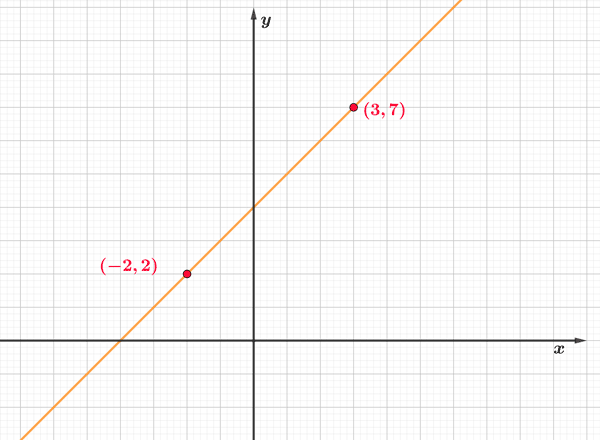$y-7=1(x-3)$ $y-7=3(x-1)$ $y-2=1(x-2)$ $y+2=1(x-2)$
•  Question 7: 2 pts Find the equation of the line graphed on the picture.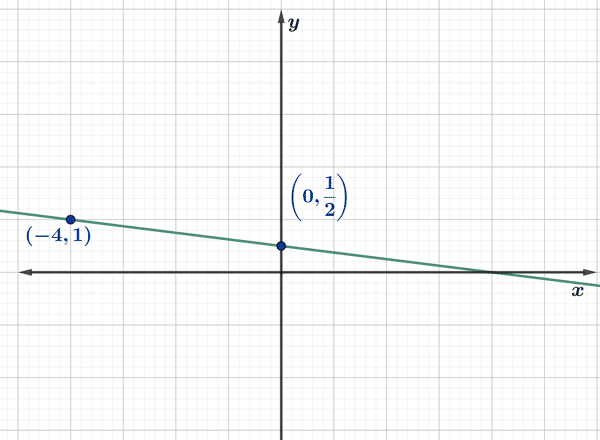•  Question 8: 2 pts Find the equation of the line graphed on the picture.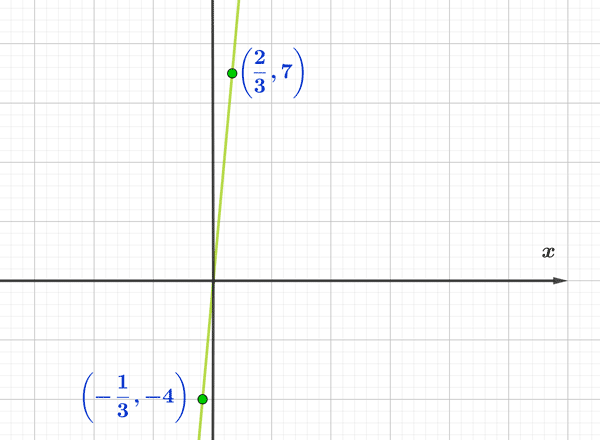$y-4=11\left(x-\dfrac{1}{3}\right)$ $y-7=11\left(x-\dfrac{2}{3}\right)$ $y-\dfrac{2}{3}=11\left(x-4\right)$ $y-7=11\left(x-\dfrac{2}{3}\right)$
•  Question 9: 3 pts Find the equation of the line graphed on the picture.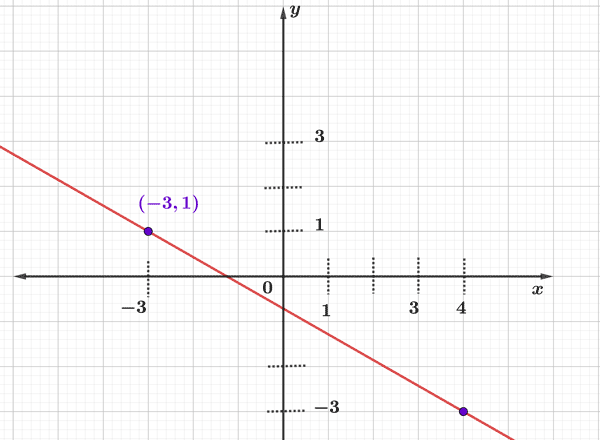$y+1=\dfrac{4}{7}\left(x-3\right)$ $y+3=\dfrac{4}{7}\left(x-1\right)$ $y+3=-\dfrac{4}{7}\left(x-4\right)$ $y-3=-\dfrac{4}{7}\left(x+4\right)$
•  Question 10: 3 pts Find the equation of the line graphed on the picture.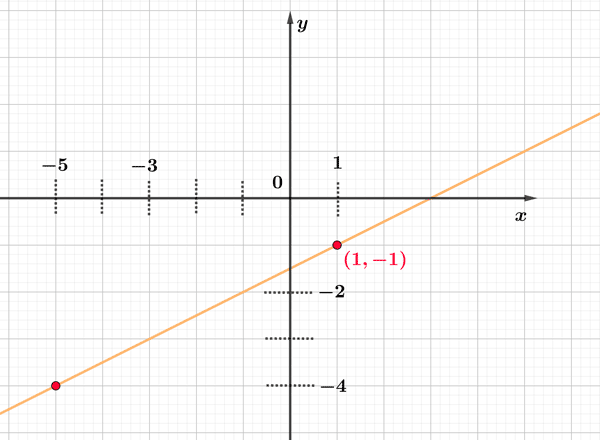•  Question 11: 3 pts Find the equation of the line graphed on the picture.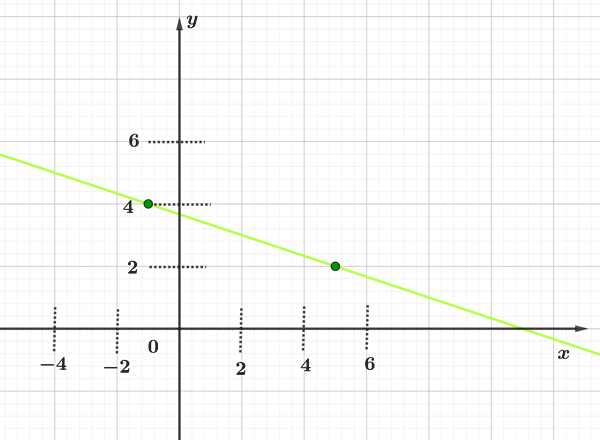$y-2=\dfrac{1}{3}\left(x-5\right)$ $y-2=-\dfrac{1}{3}\left(x-5\right)$ $y-1=-\dfrac{1}{3}\left(x-4\right)$ $y+1=\dfrac{1}{3}\left(x-4\right)$
•  Question 12: 3 pts Find the equation of the line graphed on the picture.